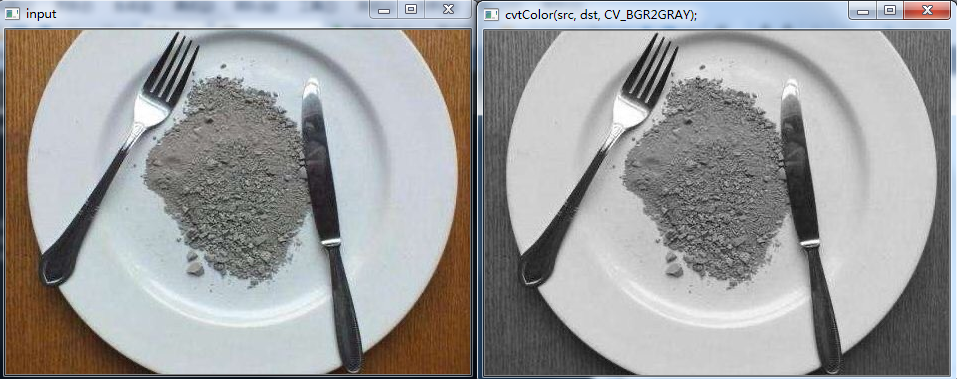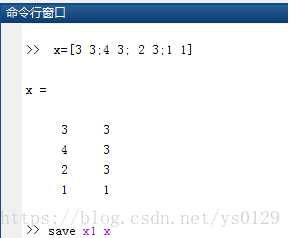• 2016-11-18 19:34:31
#include "opencv2/imgproc/imgproc.hpp"
#include "opencv2/highgui/highgui.hpp"
#include <iostream>
int main( )
{
cv::Mat image1;

cv::Mat image2( 6, 6, CV_8UC1 );

cv::Mat image3( cv::Size(7,7), CV_8UC3 );

cv::Mat image4( 8, 8, CV_32FC2, cv::Scalar(1,3) );

cv::Mat image5( cv::Size(9,9), CV_8UC3, cv::Scalar(1,2,3) );

cv::Mat image6( image2 );

std::cout << image1 << std::endl;
getchar();
std::cout << image2 << std::endl;
getchar();
std::cout << image3 << std::endl;
getchar();
std::cout << image4 << std::endl;
getchar();
std::cout << image5 << std::endl;
getchar();
std::cout << image6 << std::endl;
return 0;
}

更多相关内容
• Mat dst; dst=Mat(src.size(), src.type()); //和src图片一样大小，一样类型 dst = Scalar(127, 0, 255); namedWindow("output", CV_WINDOW_AUTOSIZE); imshow("output", dst); 克隆图片...

只用来记录学习笔记

新生成一个rgb为（127, 0, 255）的图像：

	Mat dst;
dst=Mat(src.size(), src.type()); //和src图片一样大小，一样类型
dst = Scalar(127, 0, 255);
namedWindow("output", CV_WINDOW_AUTOSIZE);
imshow("output", dst);


克隆图片方法1：

Mat dst = src.clone();
namedWindow("output", CV_WINDOW_AUTOSIZE);
imshow("output", dst);


克隆图片方法2：

Mat dst;
src.copyTo(dst);
namedWindow("output", CV_WINDOW_AUTOSIZE);
imshow("output", dst);


颜色空间转换函数：

cvtColor(src, dst, CV_BGR2GRAY); //转成灰色


转成灰色效果图：通道：

src.channels()


基本上，描述一个像素点，如果是灰度，那么只需要一个数值来描述它，就是单通道。
如果一个像素点，有RGB三种颜色来描述它，就是三通道。
windows的bmp有时候是一个四通道图像，R、G、B加上一个A通道，表示透明度。

获取图像数据指针：

const uchar* firstRow = dst.ptr<uchar>(0);


新建一个Mat：

Mat  m1;
m1.create(src.size(), src.type());
m1 = Scalar(0, 0, 255);


新建一个Mat：

Mat m2 = Mat::zeros(2,2 ,CV_8UC1);


新建Mat：

Mat C = (Mat_<double>(3, 3) << 0, -1, 0, -1, 5, -1, 0, -1, 0);

展开全文opencv
• 1、mat文件的生成(1)直接在Matlab中创建并保存矩阵数据打开Matlab软件，点击左上角文件(File)，然后点击新建(new)，选择变量(Variable)，就新建了一个mat文件。点击你新建的文件，即可打开一个编辑器，输入数据即可...

1、mat文件的生成

(1)直接在Matlab中创建并保存矩阵数据

打开Matlab软件，点击左上角文件(File)，然后点击新建(new)，选择变量(Variable)，就新建了一个mat文件。

点击你新建的文件，即可打开一个编辑器，输入数据即可。

(2)保存单一矩阵数据

如图所示，输入数据，生成数组。最后输入save x1 x。该语句表示将敲入的数组x生成数据x1.mat文件。(3)如果矩阵A.B都已赋值，现在需要将A,B矩阵保存成mat格式文件，执行如下命令：save mydata A B 就会把A B矩阵数据保存在mydata.dat文件中了。

2、mat文件的读取

(1)x1.mat文件和代码在同一个文件目录下。

(2)x1.mat文件和代码不在同一个文件目录下。

展开全文• I have 25 images in a folder and I want to make a .mat file for a road-sign recognition system.What are the steps for making a .mat file in Matlab?解决方案There may be a better approach for images but...I have 25 images in a folder and I want to make a .mat file for a road-sign recognition system.

What are the steps for making a .mat file in Matlab?

解决方案

There may be a better approach for images but here is what I know. If you want to control what goes into the .mat file you can specify what variables in your workspace will be saved using the save command.

% MATLAB R2017a

X = rand(273,273);

Y = rand(273,273);

Z = rand(273,273);

save FileName X Y Z

This creates a file FileName.mat.

You can access the contents using the load command.

clear

To save everything in the workspace to a .mat file, use the save command without specifying the variables to save (MATLAB will then save them all).

W = rand(273,273);

save FileName

See the linked documentation for more options and examples.

This requires you to loop through the images in the folder. A direct approach to this is directly loading the images using a loop over [filepath 'image' num2str(j) '.jpg'] with index j where filepath = 'C:\Users\user1\Folder\ImageFolder\'. This uses string concatenation and the num2str command.

If you need to change your current directory within the script,the cd function is useful.

展开全文• 1， #include<iostream> #include<opencv2/opencv.hpp> using namespace std; using namespace cv;... //创建一个2*2 类型为32位的浮点型三通道... Mat img(2, 2, CV_32FC3, Scalar(0, 255, 255)); cout &opencv c++
• ## c++ 创建 mat

千次阅读 2020-12-05 22:54:19
Scalar是bgr： Mat imgM(200, 200, CV_8UC3, Scalar(255, 255, 255)); cv::imshow("bar_img", imgM); cv::waitKey();
• 对于OpenCV 1.x: 您可以使用CreateMat来执行此操作：Creates a matrix header and allocates the matrix data.Python: cv.CreateMat(rows, cols, type) → mat Parameters: rows – Number of rows in the matrix ...
• 2018.10.8更新 不过最新的学习发现，如果CV::MAT中的数据是连续的，也可以把数据用memcpy拷贝到对应的data指针指向的内存中。不过需要注意的是如果不连续，这样做是不可以的。...inline Mat::Mat(int _ro...OPENCV
• opencv学习笔记之Mat类型创建 1.构造多通道的Mat类型 Mat(int rows,int cols, cv_32FC(n)) 这是常见的Mat类型创建方法 2.使用成员函数clone 和 copyTo Mat image = NewImage.rowRanag(2,4).clone(); 目的将NewImage...
• 这样就会创建一个变量名为“feature”的变量（这个变量名是可以更改的）。...同样的操作，创建元路径 这样就将两个.mat文件保存到一个文件中了...2、将多个.mat文件保存为一个.mat文件 3. 将excel文件保存为.mat文件 ...matlab
• Mat mat = new Mat(3,2, CvType.CV_32SC3); 第一二个参数是行列数，第三个参数是mat的类型。这里32SC3表示一个像素用了32位，S代表signed int，C3代表通道数是3。 即 创建一个3X2的矩阵，此矩阵具有3通道。每个点...java opencv 计算机视觉
• 参考：OpenCV：创建Mat矩阵的几种常用方法 本章介绍创建Mat的几种方式。 #include <opencv2/opencv.hpp> using namespace cv; int main() { //-----------// // Method 1: // //-----------// Mat m; ...github pytorch 深度学习
• MAT 创建图像对象的四种方法 Mat 对象的创建有多种方式：克隆、复制、赋值、构造函数。 Mat src = imread("d:/test.jpg"); //读取源地址 构造函数 Mat img_constructe(src); 赋值 Mat img_assign = ...opencv python 计算机视觉
• 第一种方法： 点击新建变量，然后复制进去 第二种方法：打开xls文件，类型选择数值矩阵，导出数据 在工作区右击生成的数据，另存为mat格式就好了matlab 开发语言
• static void Main(string[] args) ... Mat src = Cv2.ImRead("lenna.png", ImreadModes.AnyColor); Cv2.ImShow("src image", src); Mat m1 = src.Clone(); Mat m2 = new Mat(); src.CopyTo(m2); ...opencv c#
• 方法一:使用Mat（）构造函数，这种方法简单明了，可以为图像开辟内存，指定初始值等 Mat M(4,4,CV_8UC3,Scalar(0,0,255));//创建三个通道的图像，并为其定义尺寸、及通道的初始值方法二：通过构造函数进行初始化int...
• Mat () > 2. Mat (int rows, int cols, int type) > 3. Mat (Size size, int type) > 4. Mat (int rows, int cols, int type, const Scalar &s) > 5. Mat (Size size, int type, const Scalar &...
• 创建Mat空间 (1) 通过Mat 构造函数直接创建 Mat mat(3000,4000,CV_8UC3); (2) 使用mat.Create创建 mat.Create(rows,cols,CV_8UC1) Opencv 提供了isContinuous来判断存储空间是否是连续的，通过step记录 ...
• 一、参考 1、OpenCV For Unity Mat容器的创建与矩阵操作基础 https://www.jianshu.com/p/584c9d71198d ①总结：good：让我理解了一些基本的Mat知识，适合多看
• opencv(三) 基本使用 Mat矩阵的创建与初始化 1.标准矩阵的创建 2.一般矩阵的创建 3.矩阵的初始化 4.源码 1.标准矩阵的创建 输出结果: 2.一般矩阵的创建 3.矩阵的初始化 输出结果: 4...opencv matrix
• OpenCV4(C++)认识图像对象-Mat并以六种方式创建Mat，以及获取图像宽 高 通道 深度 类型，运用数组和指针遍历与访问像素opencv c++opencv 计算机视觉 机器学习 c++ python
• 1、使用Mat（）构造函数 2、在C/C++中通过构造函数进行初始化 3、为已存在的IplImage指针创建信息头 4、利用create()函数 5、采用matlab式的初始化方式: zeros(), ones(), eyes() 6、对小矩阵使用逗号分隔式...c++ 计算机视觉 opencv
• ## Mat的几种创建方法

千次阅读 2017-09-19 16:14:06
Mat mat(1,1,CV_8UC3); Mat mat = (Mat_(1, 3) ,0,0);
• Android 开发过程中为了实现代码的高效性，通常要调用本地c++代码，JNI是java语言提供的和c/c++相互...c++部分新建Mat指针指向java传入的内存区域，将处理后的结果图像的Mat数据复制到这块内存区域，这样java中的创建的
• Mat 也增加了迭代器的支持,以便于矩阵元素的遍历。 下面的例程功能跟上一节的例程类似,但是由于使用了迭代器,而不是使用行数和列数来遍历,所以这儿没有了 i 和 j 变量,图像的像素值设置为一个随机数。 #...opencv
• 创建一个Mat对象有多种方法，本文将列举最为常用的五种： 1.使用Mat()构造函数， 2.利用create()函数创建，用来为已有矩阵开辟内存，不能用来初始化 3.类似于Matlab式的初始化方式eye，zeros，ones， 4.对小型...
• //整理创建Mat的方法 //1. 使用Mat()构造函数构建 /** @overload @param rows Number of rows in a 2D array. 二维数组的行数，又图像的高度 @param cols Number of columns in a 2D array. 二维数组的列数，又...
• 下面的两种方法是我自己比较...Mat E = Mat::eye(4, 4, CV_64F); Mat O = Mat::ones(2, 2, cv_32F); Mat Z = Mat::zeros(3, 3, CV_8UC1);CV_8UC3 使用Mat()构造矩阵 Mat M(2, 2, CV_8UC3, Scalar(0, 0, 255)); ......